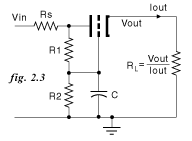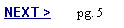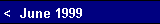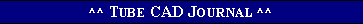Tube Regulators, part 2       John Atwood
 Series and Shunt Regulators   The two divisions of regulators described in part 1 are series and shunt regulators. Both work by comparing the output with a reference voltage and using the difference to drive a control element. The control element is basically a controllable resistance that modulates the current flow. In the case of a shunt regulator, the control element is from the output to ground, with a series dropping resistor from the source to isolate the input and output. The series regulator simply uses the control element as a variable resistor between the source and load.
 Since the grid draws very low current, a high value resistor and capacitor can be used to provide a very slow turn-on. This technique (using power MOSFETs) was used by John Nunes in the design of some recent Dynaco and MFA amplifiers. He called this a power supply "buffer". When a tube is used, the Thevenin equivalent resistance of R1||R2 should be less than the fixed-bias maximum grid resistor spec, otherwise the output voltage can drift if the tube has any residual grid current.     At frequencies several times above the time constant of R1||R2 and C, the grid of the tube is essentially stable for AC. For AC, the line regulation (AC input noise / AC output noise) is:     kr = [(rp + Rs) / RL] + mu + 1where:       rp =    plate resistance of tube (at operating point used)     Rs =   source impedance upstream from regulator      RL =  equivalent load resistance =  Vout / Iout      mu =  mu (amplification factor) of tubeThe output impedance (AC output voltage variation / AC load current variation) is:       Zo = (rp + Rs) / (mu + 1)If Rs is much less than rp, Zo is approximately:      Zo =  rp / ( mu + 1) or approximately 1/gm.   The voltage divider resistors R1 and R2 should be chosen so that the grid of the tube is less than the desired Vout by the expected bias voltage for the tube. This can be obtained from the curve of the tube. As an example, if Vin is 300V, Vout is 150V, and the load current is 15 mA, then for 1/2 of a 5687, the bias voltage would be about -6V. At this operating point, the gm is 7000 umhos, the rp is 2.5K and the mu is 17. If Rs = 0, the output impedance would then be 1/gm or 145 ohms. AC noise on the input is reduced by a factor of 18.5 or 25 dB.   The capacitor C in fig. 2.3 isolates the voltage reference from the input for AC, but the circuit has no immunity from slowly changing DC input voltage variations. A way to improve the DC and very low frequency performance of the cathode-follower regulator is to take the reference voltage divider from the output instead of the input as shown in fig. 2.4.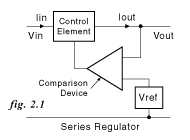Simple Series Regulators   Series regulators are the most efficient linear regulators, since all the current (excluding that needed for the reference and difference circuits) flows to the load. The only power dissipation is in the series control element (the "pass" device). The simplest series regulator uses the amplifying characteristics of the control element itself as the comparison circuit. In this case, as in fig. 2.3, the control element is a cathode follower, emitter follower, or source follower, depending on the technology used. The regulation ability is limited by the finite transconductance of the pass device, but is often sufficient for many needs.